# Maths Brain Teasers 110 :: Can You - Complete the Table?

in STEMGeekslast year

Hey All; @StemGeeks Mathematician;

Without further, ado, let's get to our puzzle for today:: Maths Brain Teasers 110 :: Can You - Complete the Table?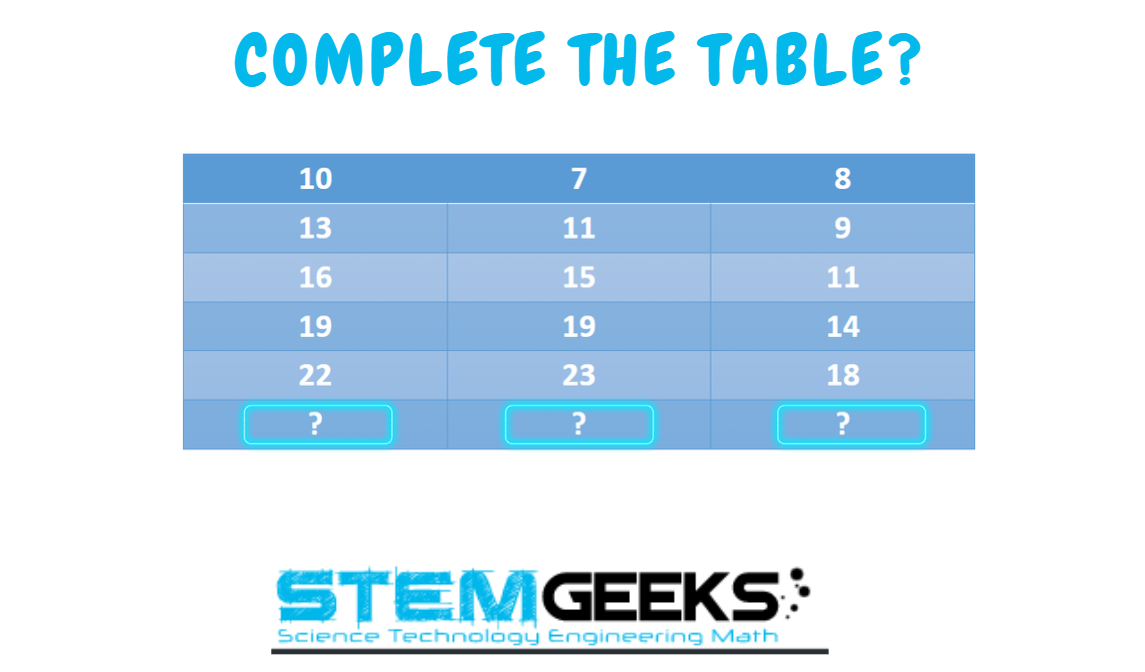Have a close look at the image given and see if you can decode the logic given in the image to complete the puzzle. Try finding out the right NUMBERs that Simplify or Decode the logic to the given puzzle. Replace the question marks with the right numbers so that it completes the series.

There is logic to resolve the puzzle; try finding out the logic and it should be resolved in seconds.. Guesswork isn't going to help...

With that, I'll leave you all with the Maths Brain Teasers 110 :: Can You - Complete the Table? Good luck solving the puzzle...

##### Maths Brain Teasers 109 :: Can You - Complete the Puzzle? - Solved with Explanation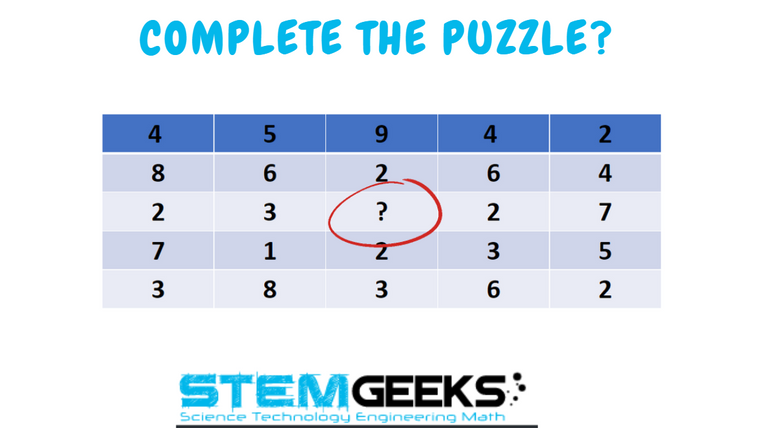Let's have a look at the Step by Step instructions to Solve the Maths Brain Teasers 109 :: Can You - Complete the Puzzle?

If you carefully look at the Image in question; You would have noticed the following pattern is being followed::

The sum of the numbers in each column decreases by one from left to right

The logic was:: The sum of numbers in each column decreases by 1 from LEFT to RIGHT for example::

Let's take the second column::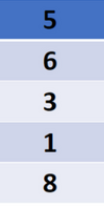### The total 0f this column is:: 23

Now, let's take the column in question::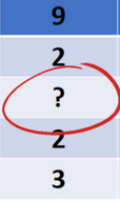As per the logic this column sum should be 22

### 9 + 2 + 6 + 2 + 3 = 22

Hence the answer to the Puzzle is Number:: 6

### STEM token GiveAway

I'll be again doing a giveaway of STEM tokens to the lucky random winner with the correct answer. For the last contest, which was Maths Brain Teasers 109 :: Can You - Complete the Puzzle?.

We had only 2 entries and I am happy to see the engagement. At the same time, it's great to see the detailed answers provided by everyone as to how they arrived at the solution of the puzzle.. Well done guys.. Way to GO... Now the results part; None of the entries provided the right answer. And hence I'll be moving the reward of this puzzle to the next puzzle; with that, the reward for this puzzle i.e. 110th puzzle would be 20 STEM tokens...

### Math Quote for the Day::

Here is the motivation to solve the Maths Equation Puzzle?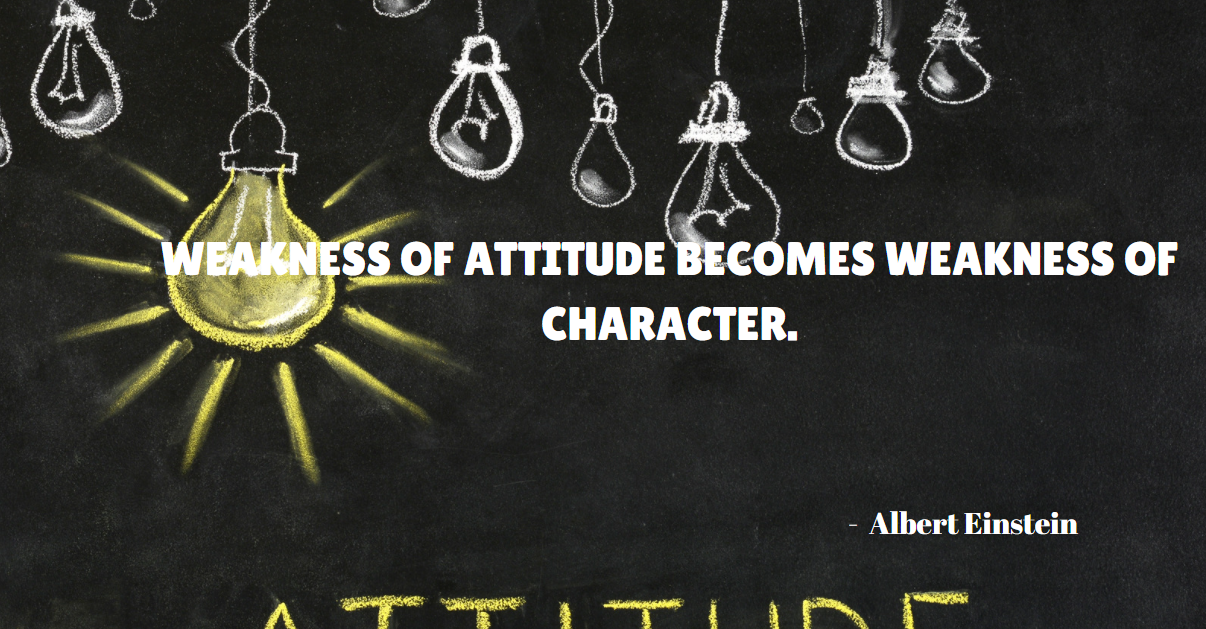If you like my work, then please spread the Word.. that we do have the Math Brain Teasers competition here @StemGeeks platform. Reblog is much appreciated.

Best RegardsFind Me on the Other Social Media Platforms::PS:: All the Maths Brain Teasers; are made by me using the Pro Canva License Version

Sort:

First column: 10, (+3) 13, (+3) 16, (+3) 19, (+3) 22, (+3) 25
Second column: 7, (+4) 11, (+4) 15, (+4) 19, (+4) 23, (+4) 27
Third column: 8, (+1) 9, (+2) 11, (+3) 14, (+4) 18, (+5) 23

last year (edited)

First column is 25 ,

Second column is 27 and

Third column is 23 respectively...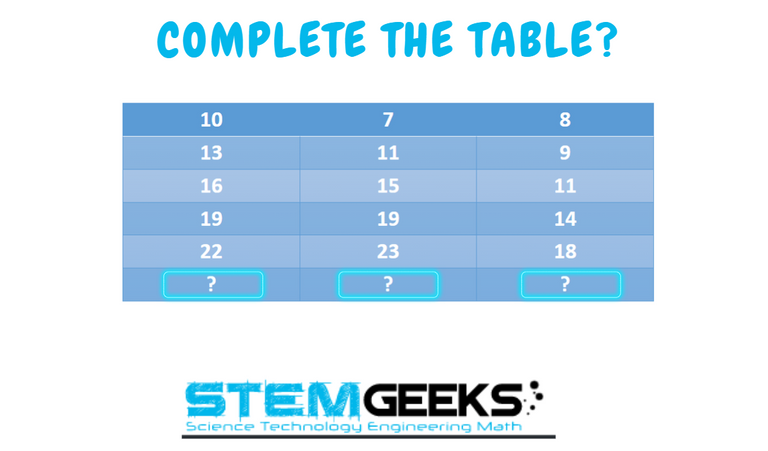For the first column, 3 is added to 10 while 4 is added to 7 in second column and 1 added 8 in the 3rd column.

The first column adds up in the progression of 3, while 7 adds up in the progression of 4.

The third column is quite unique because it's progression increases by an addition of 1 for every number added to it.

8 + 1 = 9
9 + 2 = 11
11 + 3 = 14
14 + 4 = 18

Therefore, 18 + 5 = 23.

For the three columns, we have a final answer of 24, 27 and 23.

### Solution

The first pot has an addition of 3
The second pot has an addition of 4
While the third pot varies in addition with a +1,+2,+3,+4 and +5 respectively.
The answer is 25, 27 and 23.

Answer is 27, 25 and 23
!LUV 1